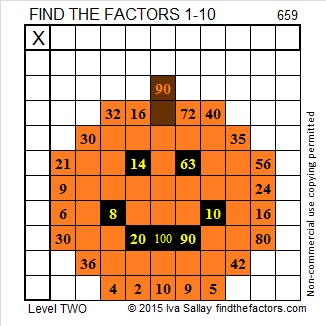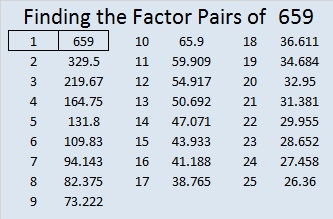# 659 Jack O’lantern Puzzle

659 is the sum of the 7 prime numbers from 79 to 107. Can you list them all before you add them up?

Enjoy this Jack O’lantern puzzle.Print the puzzles or type the solution on this excel file: 10 Factors 2015-10-26

———————————————

• 659 is a prime number.
• Prime factorization: 659 is prime.
• The exponent of prime number 659 is 1. Adding 1 to that exponent we get (1 + 1) = 2. Therefore 659 has exactly 2 factors.
• Factors of 659: 1, 659
• Factor pairs: 659 = 1 x 659
• 659 has no square factors that allow its square root to be simplified. √659 ≈ 25.670995.How do we know that 659 is a prime number? If 659 were not a prime number, then it would be divisible by at least one prime number less than or equal to √659 ≈ 25.7. Since 659 cannot be divided evenly by 2, 3, 5, 7, 11, 13, 17, 19, or 23, we know that 659 is a prime number.

———————————

Since riccardo took the time to photograph the puzzle’s solution, I decided to include it in this post as well. Great job riccardo!

1.Joseph Nebus
•ivasallay